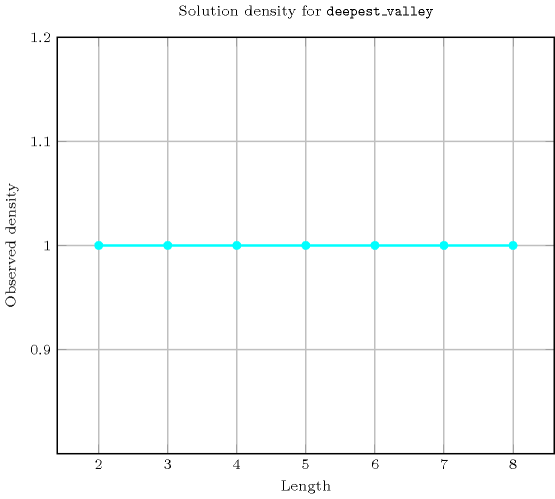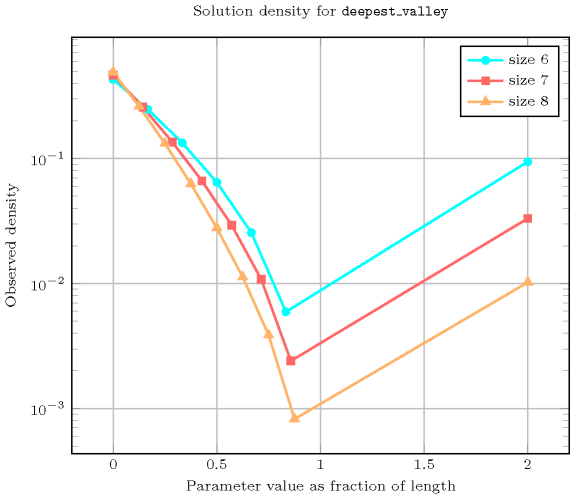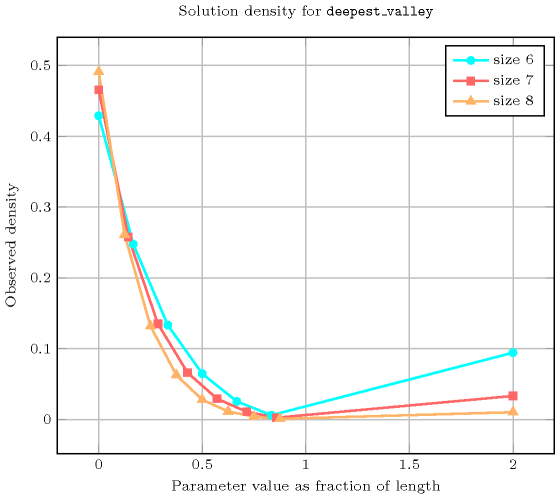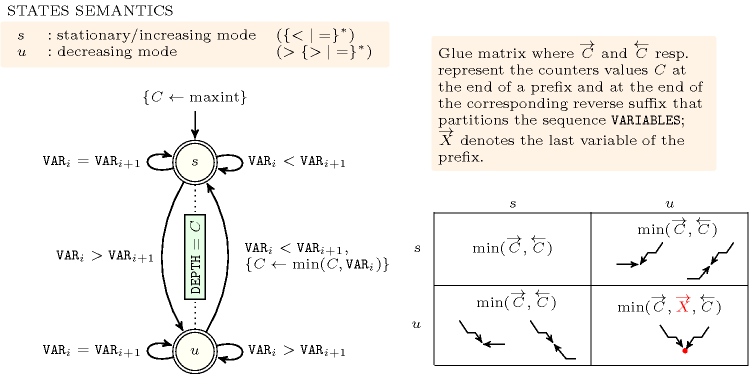## 5.113. deepest_valley

Origin

Derived from $\mathrm{𝚟𝚊𝚕𝚕𝚎𝚢}$.

Constraint

$\mathrm{𝚍𝚎𝚎𝚙𝚎𝚜𝚝}_\mathrm{𝚟𝚊𝚕𝚕𝚎𝚢}\left(\mathrm{𝙳𝙴𝙿𝚃𝙷},\mathrm{𝚅𝙰𝚁𝙸𝙰𝙱𝙻𝙴𝚂}\right)$

Arguments
 $\mathrm{𝙳𝙴𝙿𝚃𝙷}$ $\mathrm{𝚍𝚟𝚊𝚛}$ $\mathrm{𝚅𝙰𝚁𝙸𝙰𝙱𝙻𝙴𝚂}$ $\mathrm{𝚌𝚘𝚕𝚕𝚎𝚌𝚝𝚒𝚘𝚗}\left(\mathrm{𝚟𝚊𝚛}-\mathrm{𝚍𝚟𝚊𝚛}\right)$
Restriction
$\mathrm{𝚛𝚎𝚚𝚞𝚒𝚛𝚎𝚍}$$\left(\mathrm{𝚅𝙰𝚁𝙸𝙰𝙱𝙻𝙴𝚂},\mathrm{𝚟𝚊𝚛}\right)$
Purpose

A variable ${V}_{k}$ $\left(1 of the sequence of variables $\mathrm{𝚅𝙰𝚁𝙸𝙰𝙱𝙻𝙴𝚂}={V}_{1},\cdots ,{V}_{m}$ is a valley if and only if there exists an $i$ $\left(1 such that ${V}_{i-1}>{V}_{i}$ and ${V}_{i}={V}_{i+1}=\cdots ={V}_{k}$ and ${V}_{k}<{V}_{k+1}$. $\mathrm{𝙳𝙴𝙿𝚃𝙷}$ is the minimum value of the valley variables. If no such variable exists $\mathrm{𝙳𝙴𝙿𝚃𝙷}$ is equal to the default value $\mathrm{𝙼𝙰𝚇𝙸𝙽𝚃}$.

Example
 $\left(2,〈5,3,4,8,8,2,7,1〉\right)$ $\left(7,〈1,3,4,8,8,8,7,8〉\right)$

The first $\mathrm{𝚍𝚎𝚎𝚙𝚎𝚜𝚝}_\mathrm{𝚟𝚊𝚕𝚕𝚎𝚢}$ constraint holds since 2 is the deepest valley of the sequence $53488271$.

##### Figure 5.113.1. Illustration of the first example of the Example slot: a sequence of eight variables ${V}_{1}$, ${V}_{2}$, ${V}_{3}$, ${V}_{4}$, ${V}_{5}$, ${V}_{6}$, ${V}_{7}$, ${V}_{8}$ respectively fixed to values 5, 3, 4, 8, 8, 2, 7, 1 and its corresponding deepest valley of depth 2Typical
 $|\mathrm{𝚅𝙰𝚁𝙸𝙰𝙱𝙻𝙴𝚂}|>2$ $\mathrm{𝚛𝚊𝚗𝚐𝚎}$$\left(\mathrm{𝚅𝙰𝚁𝙸𝙰𝙱𝙻𝙴𝚂}.\mathrm{𝚟𝚊𝚛}\right)>2$ $\mathrm{𝚟𝚊𝚕𝚕𝚎𝚢}$$\left(\mathrm{𝚅𝙰𝚁𝙸𝙰𝙱𝙻𝙴𝚂}.\mathrm{𝚟𝚊𝚛}\right)>0$
Symmetry

Items of $\mathrm{𝚅𝙰𝚁𝙸𝙰𝙱𝙻𝙴𝚂}$ can be reversed.

Arg. properties

Functional dependency: $\mathrm{𝙳𝙴𝙿𝚃𝙷}$ determined by $\mathrm{𝚅𝙰𝚁𝙸𝙰𝙱𝙻𝙴𝚂}$.

Counting
 Length ($n$) 2 3 4 5 6 7 8 Solutions 9 64 625 7776 117649 2097152 43046721

Number of solutions for $\mathrm{𝚍𝚎𝚎𝚙𝚎𝚜𝚝}_\mathrm{𝚟𝚊𝚕𝚕𝚎𝚢}$: domains $0..n$Length ($n$)2345678
Total9646257776117649209715243046721
 Parameter value

0-917629005047297622721133632
1-49917122912554057611233250
2-144900156802832505665896
3--1138075871385442693425
4---923000613891195056
5----69722632484020
6-----5036166208
7------35443
100000095029517921108869498439791

Solution count for $\mathrm{𝚍𝚎𝚎𝚙𝚎𝚜𝚝}_\mathrm{𝚟𝚊𝚕𝚕𝚎𝚢}$: domains $0..n$Figure 5.113.2 depicts the automaton associated with the $\mathrm{𝚍𝚎𝚎𝚙𝚎𝚜𝚝}_\mathrm{𝚟𝚊𝚕𝚕𝚎𝚢}$ constraint. To each pair of consecutive variables $\left({\mathrm{𝚅𝙰𝚁}}_{i},{\mathrm{𝚅𝙰𝚁}}_{i+1}\right)$ of the collection $\mathrm{𝚅𝙰𝚁𝙸𝙰𝙱𝙻𝙴𝚂}$ corresponds a signature variable ${S}_{i}$. The following signature constraint links ${\mathrm{𝚅𝙰𝚁}}_{i}$, ${\mathrm{𝚅𝙰𝚁}}_{i+1}$ and ${S}_{i}$:
${\mathrm{𝚅𝙰𝚁}}_{i}<{\mathrm{𝚅𝙰𝚁}}_{i+1}⇔{S}_{i}=0\wedge {\mathrm{𝚅𝙰𝚁}}_{i}={\mathrm{𝚅𝙰𝚁}}_{i+1}⇔{S}_{i}=1\wedge {\mathrm{𝚅𝙰𝚁}}_{i}>{\mathrm{𝚅𝙰𝚁}}_{i+1}⇔{S}_{i}=2$.
##### Figure 5.113.2. Automaton of the $\mathrm{𝚍𝚎𝚎𝚙𝚎𝚜𝚝}_\mathrm{𝚟𝚊𝚕𝚕𝚎𝚢}$ constraint and its glue matrix (state $s$ means that we are in increasing or stationary mode, state $u$ means that we are in decreasing mode, a new valley is detected each time we switch from decreasing to increasing mode and the counter $C$ is updated accordingly); $\text{maxint}$ is the largest integer that can be represented on a machine##### Figure 5.113.3. Hypergraph of the reformulation corresponding to the automaton of the $\mathrm{𝚍𝚎𝚎𝚙𝚎𝚜𝚝}_\mathrm{𝚟𝚊𝚕𝚕𝚎𝚢}$ constraint (${C}_{0}$ is set to $\text{maxint}$ the largest integer that can be represented on a machine)